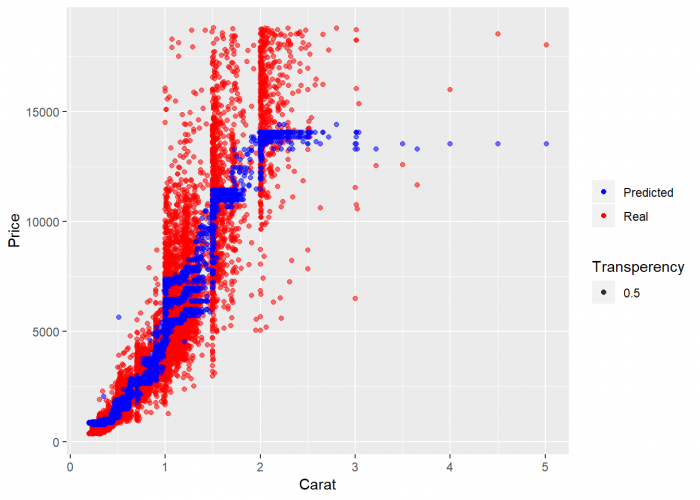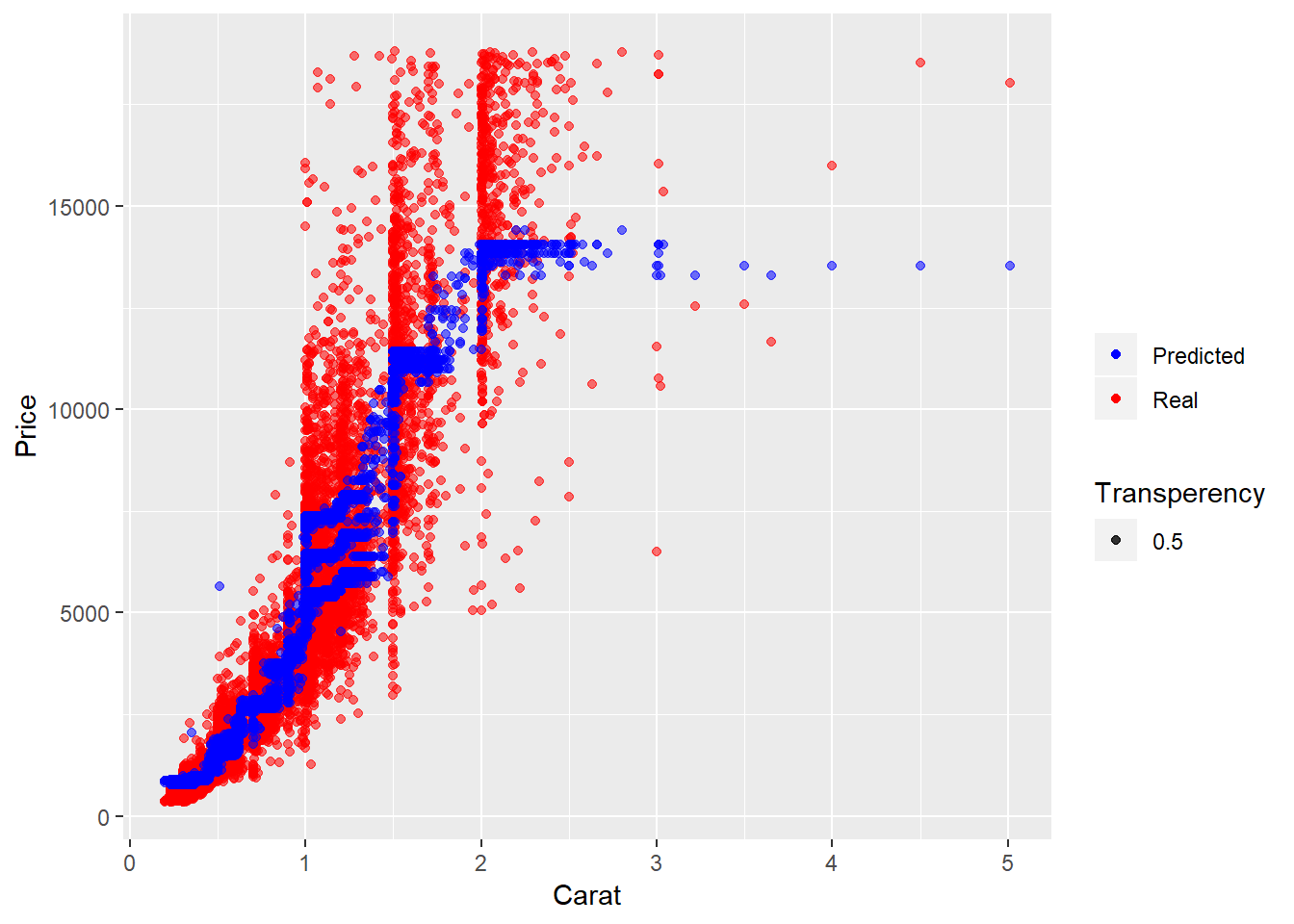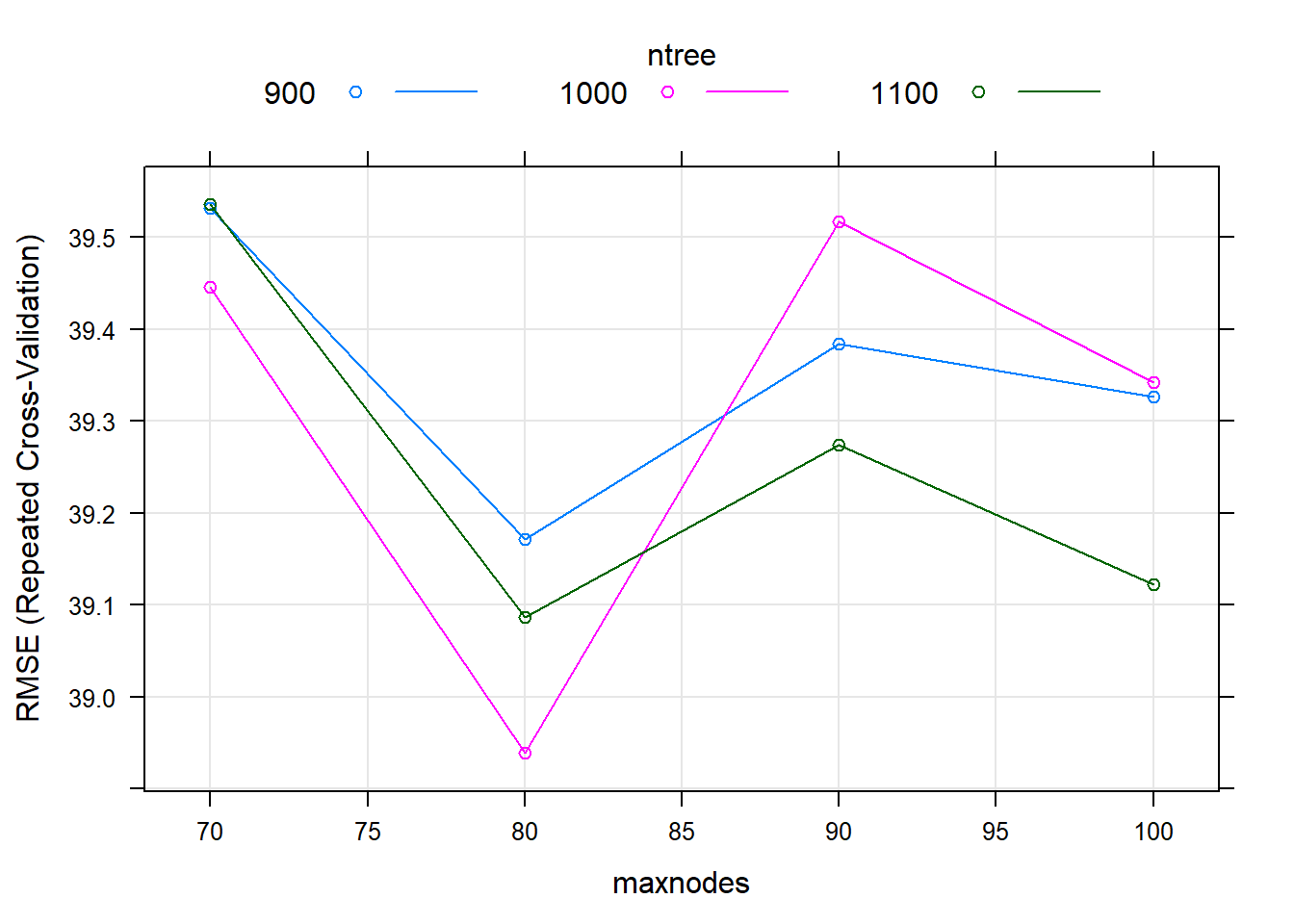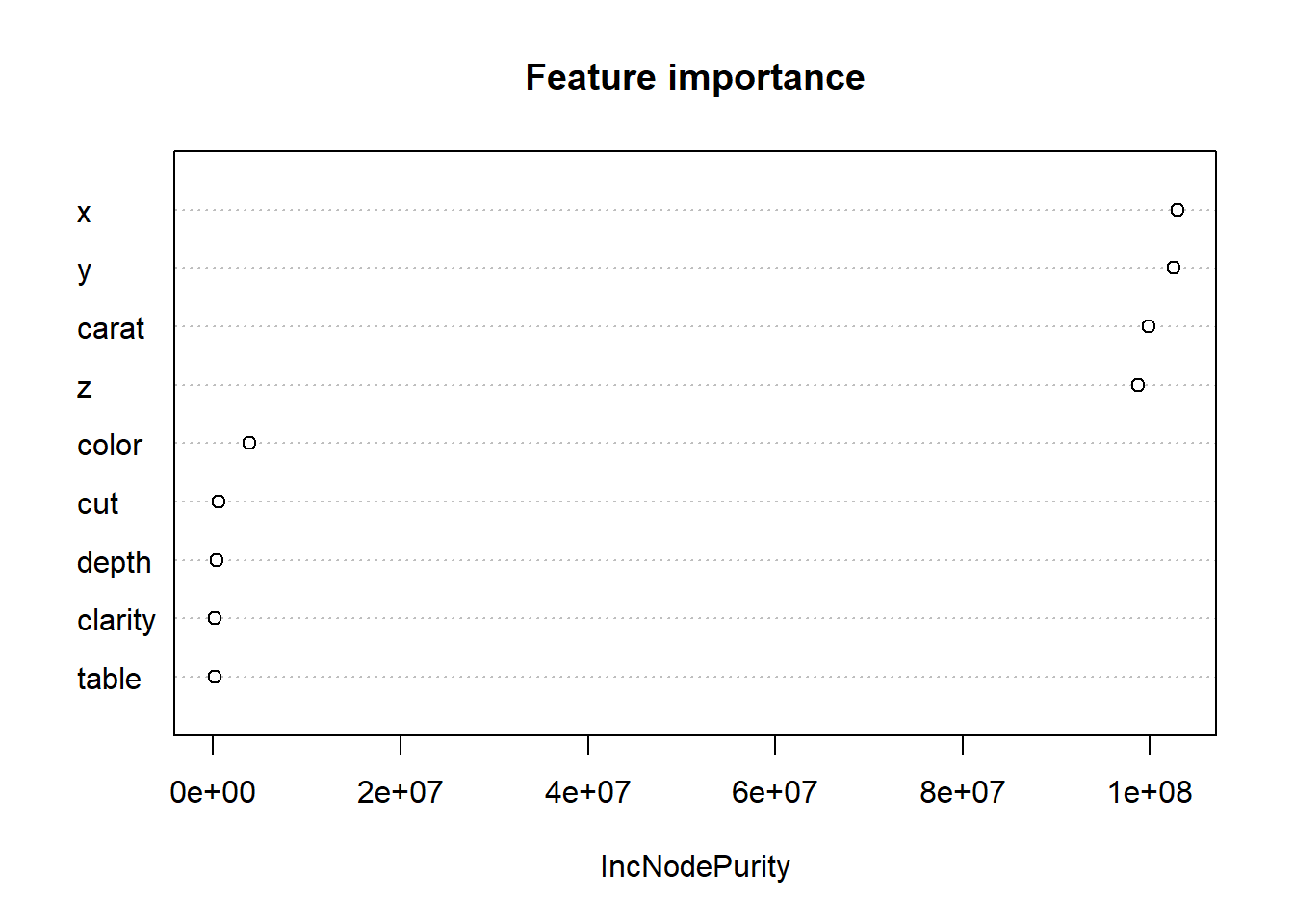# Random Forest in R: An ExampleRandom Forest is a powerful ensemble learning method that can be applied to various prediction tasks, in particular classification and regression. The method uses an ensemble of decision trees as a basis and therefore has all advantages of decision trees, such as high accuracy, easy usage, and no necessity of scaling data. Moreover, it also has a very important additional benefit, namely perseverance to overfitting (unlike simple decision trees) as the trees are combined.

In this tutorial, we will try to predict the value of diamonds from the Diamonds dataset (part of ggplot2) applying a Random Forest Regressor in R. We further visualize and analyze the obtained predictive model and look into the tuning of hyperparameters and the importance of available features.# Import the dataset
diamond <-diamonds
head(diamond)
## # A tibble: 6 x 10
##   carat cut       color clarity depth table price     x     y     z
##   <dbl> <ord>     <ord> <ord>   <dbl> <dbl> <int> <dbl> <dbl> <dbl>
## 1 0.23  Ideal     E     SI2      61.5    55   326  3.95  3.98  2.43
## 2 0.21  Premium   E     SI1      59.8    61   326  3.89  3.84  2.31
## 3 0.23  Good      E     VS1      56.9    65   327  4.05  4.07  2.31
## 4 0.290 Premium   I     VS2      62.4    58   334  4.2   4.23  2.63
## 5 0.31  Good      J     SI2      63.3    58   335  4.34  4.35  2.75
## 6 0.24  Very Good J     VVS2     62.8    57   336  3.94  3.96  2.48

The dataset contains information on 54,000 diamonds. It contains the price as well as 9 other attributes. Some features are in the text format, and we need to encode them to the numerical format. Let’s also drop the unnamed index column.

# Convert the variables to numerical
diamond$cut <- as.integer(diamond$cut)
diamond$color <-as.integer(diamond$color)
diamond$clarity <- as.integer(diamond$clarity)

head(diamond)
## # A tibble: 6 x 10
##   carat   cut color clarity depth table price     x     y     z
##   <dbl> <int> <int>   <int> <dbl> <dbl> <int> <dbl> <dbl> <dbl>
## 1 0.23      5     2       2  61.5    55   326  3.95  3.98  2.43
## 2 0.21      4     2       3  59.8    61   326  3.89  3.84  2.31
## 3 0.23      2     2       5  56.9    65   327  4.05  4.07  2.31
## 4 0.290     4     6       4  62.4    58   334  4.2   4.23  2.63
## 5 0.31      2     7       2  63.3    58   335  4.34  4.35  2.75
## 6 0.24      3     7       6  62.8    57   336  3.94  3.96  2.48

As we already mentioned, one of the benefits of the Random Forest algorithm is that it doesn’t require data scaling. So, to use this algorithm, we only need to define features and the target that we are trying to predict. We could potentially create numerous features by combining the available attributes. For simplicity, we will not do that now. If you are trying to build the most accurate model, feature creation is definitely a key part and substantial time should be invested in creating features (e.g. through interaction).

# Create features and target
X <- diamond %>%
select(carat, depth, table, x, y, z, clarity, cut, color)
geom_point( aes(x = X_test$carat , y = predictions, color = 'blue', alpha = 0.5)) + labs(x = "Carat", y = "Price", color = "", alpha = 'Transperency') + scale_color_manual(labels = c( "Predicted", "Real"), values = c("blue", "red"))The figure displays that predicted prices (blue scatters) coincide well with the real ones (red scatters), especially in the region of small carat values. But to estimate our model more precisely, we will look at Mean absolute error (MAE), Mean squared error (MSE), and R-squared scores. # Import library for Metrics library(Metrics) ## ## Attaching package: 'Metrics' ## The following objects are masked from 'package:caret': ## ## precision, recall print(paste0('MAE: ' , mae(y_test,predictions) )) ##  "MAE: 742.401258870433" print(paste0('MSE: ' ,caret::postResample(predictions , y_test)['RMSE']^2 )) ##  "MSE: 1717272.6547428" print(paste0('R2: ' ,caret::postResample(predictions , y_test)['Rsquared'] )) ##  "R2: 0.894548902990278" We obtain high error values (MAE and MSE). To improve the predictive power of the model, we should tune the hyperparameters of the algorithm. We can do this manually, but it will take a lot of time. In order to tune the parameters ntrees (number of trees in the forest) and maxnodes (maximum number of terminal nodes trees in the forest can have), we will need to build a custom Random Forest model to obtain the best set of parameters for our model and compare the output for various combinations of the parameters. ### Tuning the parameters # If training the model takes too long try setting up lower value of N N=500 #length(X_train) X_train_ = X_train[1:N , ] y_train_ = y_train[1:N] seed <-7 metric<-'RMSE' customRF <- list(type = "Regression", library = "randomForest", loop = NULL) customRF$parameters <- data.frame(parameter = c("maxnodes", "ntree"), class = rep("numeric", 2), label = c("maxnodes", "ntree"))

customRF$grid <- function(x, y, len = NULL, search = "grid") {} customRF$fit <- function(x, y, wts, param, lev, last, weights, classProbs, ...) {
randomForest(x, y, maxnodes = param$maxnodes, ntree=param$ntree, ...)
}

customRF$predict <- function(modelFit, newdata, preProc = NULL, submodels = NULL) predict(modelFit, newdata) customRF$prob <- function(modelFit, newdata, preProc = NULL, submodels = NULL)
predict(modelFit, newdata, type = "prob")
customRF$sort <- function(x) x[order(x[,1]),] customRF$levels <- function(x) x$classes # Set grid search parameters control <- trainControl(method="repeatedcv", number=10, repeats=3, search='grid') # Outline the grid of parameters tunegrid <- expand.grid(.maxnodes=c(70,80,90,100), .ntree=c(900, 1000, 1100)) set.seed(seed) # Train the model rf_gridsearch <- train(x=X_train_, y=y_train_, method=customRF, metric=metric, tuneGrid=tunegrid, trControl=control) Let’s visualize the impact of tuned parameters on RMSE. The plot shows how the model’s performance develops with different variations of the parameters. For values maxnodes: 80 and ntree: 900, the model seems to perform best. We would now use these parameters in the final model. plot(rf_gridsearch)Best parameters: rf_gridsearch$bestTune
##   maxnodes ntree
## 5       80  1000

### Defining and visualizing variables importance

For this algorithm, we used all available diamond features, but some of them contain more predictive power than others.

Let’s build the plot with features list on the y axis. On the X axis we’ll have incremental decrease in node impurities from splitting on the variable, averaged over all trees, it is measured by the residual sum of squares and therefore gives us a rough idea about the predictive power of the feature. Generally, it is important to keep in mind, that random forest does not allow for any causal interpretation.

varImpPlot(rf_gridsearch\$finalModel, main ='Feature importance')From the figure above you can see that the size of diamond (x,y,z refer to length, width, depth) and the weight (carat) contain the major part of the predictive power.